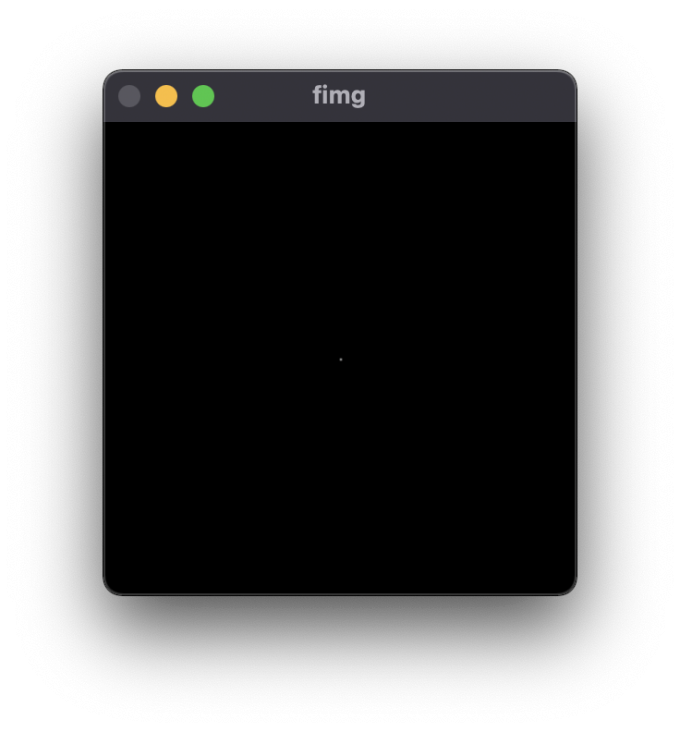# 信号图像处理：傅立叶变换与频域分析

## 傅立叶变换

wiki上用了一张图相当形象地展示了这一过程：$\hat{f}(\xi)=\int^\infty_{-\infty}f(x)\exp{(-2\pi ix\xi)}\text{d}x$

### 离散傅立叶变换

$\hat{x}[k] = \sum^{N-1}_{n = 0}\exp{(-i\frac{2\pi}{N}nk)x[n]}$

## 图像的傅立叶变换

$F(u, v) = \sum^{M-1}_{x=0}\sum^{N-1}_{y=0}f(x,y)\exp{(-j2\pi(ux/M+vy/N))}$

$\exp{(-j2\pi(ux/M+vy/N))} = \cos\theta + j\sin\theta, \theta = 2\pi(ux/M+vy/N)$

### 离散二维傅立叶变换的代码实现- 频域图像有黑色噪声分布：图像本身有不连续的噪声出现，是缩放导致的图片噪声，将图片放大后消失## 图像频域分析的应用

### DCT隐写（两点法）

DCT隐写算法直接针对JPEG图像进行（JPEG图像天然以8x8的子块存储），隐写算法的过程如下：

1. 取出一个8x8的分块
2. 通过DCT将分块变换到频域空间
3. 随机（全局一致）选取两个点，并且根据需要嵌入的比特，通过像素值值赋予这两个点顺序（如果嵌入0则这两个点必须小的在前面，否则大的在前面，通过交换这两个像素来实现）
4. 通过IDCT将块变换回去

1. 对于每个8x8的分块做DCT
2. 根据值大小，检查是否存在交换的现象，提取该分块的比特值
3. 合并信息

Carbene Hu

2022-02-24

2022-05-12